Friday 22nd January 2021

CBSE Guess > Papers > Question Papers > Class XII > 2004 > Maths > Delhi Set-II

MATHS (Set II—Delhi)

Except for the following questions, all the remaining questions have been asked in Set I .

SECTION - A

Q. 1. 3 cards are drawn at random from a pack of well shuffled 52 cards. Find the probability that
(i) all the three cards are of the same suit;
(ii) one is a king, the other is a queen and the third is a jack.

Q. 3. Using properties of determinants show that: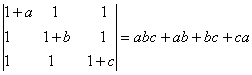Q. 5. Evaluate: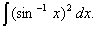Q. 10. Show that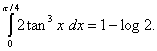Q. 14. Find the equations of the tangent and the normal to the curve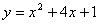at the point whose coordinate is 3.

Or

Find the intervals in which the function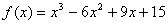(a) increasing (b) decreasing.

Q. 15. Prove that: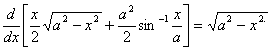Q. 17. Using matrix method solve the following system f linear equations: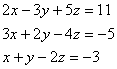SECTION - B

Q. 19. If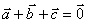show that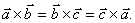SECTION - C

Q. 21. The mean and variance of a binomial distribution are 4/3 and 8/9 respectively.
Find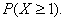Or

If the sum of the mean and variance of a binomial distribution for 6 trials be 10/3, find the distribution.

 Maths 2004 Question Papers Class XII Delhi Outside Delhi Compartment Delhi Compartment Outside DelhiSet ISet ISet ISet ISet IISet IISet IISet IISet IIISet III

CBSE 2004 Question Papers Class XII